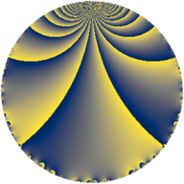# Properties

 Label 72.2.d.aLevel $72$ Weight $2$ Character orbit 72.d Analytic conductor $0.575$ Analytic rank $0$ Dimension $2$ CM discriminant -24 Inner twists $4$

# Related objects

## Newspace parameters

 Level: $$N$$ $$=$$ $$72 = 2^{3} \cdot 3^{2}$$ Weight: $$k$$ $$=$$ $$2$$ Character orbit: $$[\chi]$$ $$=$$ 72.d (of order $$2$$, degree $$1$$, minimal)

## Newform invariants

 Self dual: no Analytic conductor: $$0.574922894553$$ Analytic rank: $$0$$ Dimension: $$2$$ Coefficient field: $$\Q(\sqrt{-2})$$ Defining polynomial: $$x^{2} + 2$$ Coefficient ring: $$\Z[a_1, a_2]$$ Coefficient ring index: $$1$$ Twist minimal: yes Sato-Tate group: $\mathrm{U}(1)[D_{2}]$

## $q$-expansion

Coefficients of the $$q$$-expansion are expressed in terms of $$\beta = \sqrt{-2}$$. We also show the integral $$q$$-expansion of the trace form.

 $$f(q)$$ $$=$$ $$q + \beta q^{2} -2 q^{4} + 2 \beta q^{5} + 2 q^{7} -2 \beta q^{8} +O(q^{10})$$ $$q + \beta q^{2} -2 q^{4} + 2 \beta q^{5} + 2 q^{7} -2 \beta q^{8} -4 q^{10} -4 \beta q^{11} + 2 \beta q^{14} + 4 q^{16} -4 \beta q^{20} + 8 q^{22} -3 q^{25} -4 q^{28} + 2 \beta q^{29} -10 q^{31} + 4 \beta q^{32} + 4 \beta q^{35} + 8 q^{40} + 8 \beta q^{44} -3 q^{49} -3 \beta q^{50} -10 \beta q^{53} + 16 q^{55} -4 \beta q^{56} -4 q^{58} + 8 \beta q^{59} -10 \beta q^{62} -8 q^{64} -8 q^{70} + 14 q^{73} -8 \beta q^{77} -10 q^{79} + 8 \beta q^{80} -4 \beta q^{83} -16 q^{88} + 2 q^{97} -3 \beta q^{98} +O(q^{100})$$ $$\operatorname{Tr}(f)(q)$$ $$=$$ $$2q - 4q^{4} + 4q^{7} + O(q^{10})$$ $$2q - 4q^{4} + 4q^{7} - 8q^{10} + 8q^{16} + 16q^{22} - 6q^{25} - 8q^{28} - 20q^{31} + 16q^{40} - 6q^{49} + 32q^{55} - 8q^{58} - 16q^{64} - 16q^{70} + 28q^{73} - 20q^{79} - 32q^{88} + 4q^{97} + O(q^{100})$$

## Character values

We give the values of $$\chi$$ on generators for $$\left(\mathbb{Z}/72\mathbb{Z}\right)^\times$$.

 $$n$$ $$37$$ $$55$$ $$65$$ $$\chi(n)$$ $$-1$$ $$1$$ $$1$$

## Embeddings

For each embedding $$\iota_m$$ of the coefficient field, the values $$\iota_m(a_n)$$ are shown below.

For more information on an embedded modular form you can click on its label.

Label $$\iota_m(\nu)$$ $$a_{2}$$ $$a_{3}$$ $$a_{4}$$ $$a_{5}$$ $$a_{6}$$ $$a_{7}$$ $$a_{8}$$ $$a_{9}$$ $$a_{10}$$
37.1
 − 1.41421i 1.41421i
1.41421i 0 −2.00000 2.82843i 0 2.00000 2.82843i 0 −4.00000
37.2 1.41421i 0 −2.00000 2.82843i 0 2.00000 2.82843i 0 −4.00000
 $$n$$: e.g. 2-40 or 990-1000 Significant digits: Format: Complex embeddings Normalized embeddings Satake parameters Satake angles

## Inner twists

Char Parity Ord Mult Type
1.a even 1 1 trivial
24.h odd 2 1 CM by $$\Q(\sqrt{-6})$$
3.b odd 2 1 inner
8.b even 2 1 inner

## Twists

By twisting character orbit
Char Parity Ord Mult Type Twist Min Dim
1.a even 1 1 trivial 72.2.d.a 2
3.b odd 2 1 inner 72.2.d.a 2
4.b odd 2 1 288.2.d.a 2
5.b even 2 1 1800.2.k.e 2
5.c odd 4 2 1800.2.d.n 4
8.b even 2 1 inner 72.2.d.a 2
8.d odd 2 1 288.2.d.a 2
9.c even 3 2 648.2.n.h 4
9.d odd 6 2 648.2.n.h 4
12.b even 2 1 288.2.d.a 2
15.d odd 2 1 1800.2.k.e 2
15.e even 4 2 1800.2.d.n 4
16.e even 4 2 2304.2.a.q 2
16.f odd 4 2 2304.2.a.y 2
20.d odd 2 1 7200.2.k.h 2
20.e even 4 2 7200.2.d.p 4
24.f even 2 1 288.2.d.a 2
24.h odd 2 1 CM 72.2.d.a 2
36.f odd 6 2 2592.2.r.i 4
36.h even 6 2 2592.2.r.i 4
40.e odd 2 1 7200.2.k.h 2
40.f even 2 1 1800.2.k.e 2
40.i odd 4 2 1800.2.d.n 4
40.k even 4 2 7200.2.d.p 4
48.i odd 4 2 2304.2.a.q 2
48.k even 4 2 2304.2.a.y 2
60.h even 2 1 7200.2.k.h 2
60.l odd 4 2 7200.2.d.p 4
72.j odd 6 2 648.2.n.h 4
72.l even 6 2 2592.2.r.i 4
72.n even 6 2 648.2.n.h 4
72.p odd 6 2 2592.2.r.i 4
120.i odd 2 1 1800.2.k.e 2
120.m even 2 1 7200.2.k.h 2
120.q odd 4 2 7200.2.d.p 4
120.w even 4 2 1800.2.d.n 4

By twisted newform orbit
Twist Min Dim Char Parity Ord Mult Type
72.2.d.a 2 1.a even 1 1 trivial
72.2.d.a 2 3.b odd 2 1 inner
72.2.d.a 2 8.b even 2 1 inner
72.2.d.a 2 24.h odd 2 1 CM
288.2.d.a 2 4.b odd 2 1
288.2.d.a 2 8.d odd 2 1
288.2.d.a 2 12.b even 2 1
288.2.d.a 2 24.f even 2 1
648.2.n.h 4 9.c even 3 2
648.2.n.h 4 9.d odd 6 2
648.2.n.h 4 72.j odd 6 2
648.2.n.h 4 72.n even 6 2
1800.2.d.n 4 5.c odd 4 2
1800.2.d.n 4 15.e even 4 2
1800.2.d.n 4 40.i odd 4 2
1800.2.d.n 4 120.w even 4 2
1800.2.k.e 2 5.b even 2 1
1800.2.k.e 2 15.d odd 2 1
1800.2.k.e 2 40.f even 2 1
1800.2.k.e 2 120.i odd 2 1
2304.2.a.q 2 16.e even 4 2
2304.2.a.q 2 48.i odd 4 2
2304.2.a.y 2 16.f odd 4 2
2304.2.a.y 2 48.k even 4 2
2592.2.r.i 4 36.f odd 6 2
2592.2.r.i 4 36.h even 6 2
2592.2.r.i 4 72.l even 6 2
2592.2.r.i 4 72.p odd 6 2
7200.2.d.p 4 20.e even 4 2
7200.2.d.p 4 40.k even 4 2
7200.2.d.p 4 60.l odd 4 2
7200.2.d.p 4 120.q odd 4 2
7200.2.k.h 2 20.d odd 2 1
7200.2.k.h 2 40.e odd 2 1
7200.2.k.h 2 60.h even 2 1
7200.2.k.h 2 120.m even 2 1

## Hecke kernels

This newform subspace can be constructed as the kernel of the linear operator $$T_{5}^{2} + 8$$ acting on $$S_{2}^{\mathrm{new}}(72, [\chi])$$.

## Hecke characteristic polynomials

$p$ $F_p(T)$
$2$ $$2 + T^{2}$$
$3$ $$T^{2}$$
$5$ $$8 + T^{2}$$
$7$ $$( -2 + T )^{2}$$
$11$ $$32 + T^{2}$$
$13$ $$T^{2}$$
$17$ $$T^{2}$$
$19$ $$T^{2}$$
$23$ $$T^{2}$$
$29$ $$8 + T^{2}$$
$31$ $$( 10 + T )^{2}$$
$37$ $$T^{2}$$
$41$ $$T^{2}$$
$43$ $$T^{2}$$
$47$ $$T^{2}$$
$53$ $$200 + T^{2}$$
$59$ $$128 + T^{2}$$
$61$ $$T^{2}$$
$67$ $$T^{2}$$
$71$ $$T^{2}$$
$73$ $$( -14 + T )^{2}$$
$79$ $$( 10 + T )^{2}$$
$83$ $$32 + T^{2}$$
$89$ $$T^{2}$$
$97$ $$( -2 + T )^{2}$$